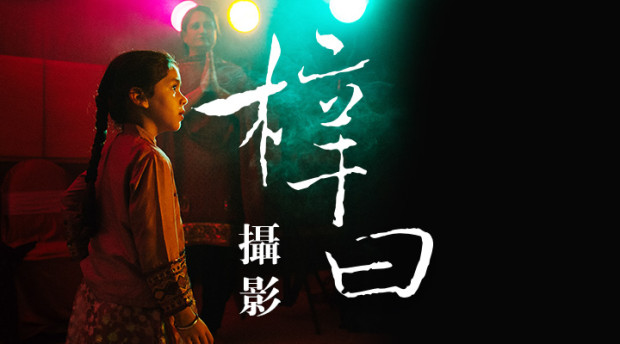# 决定摄影成功的最终要素

2014 年 4 月 10 日6月 20th, 2014No CommentsA B C D E F G H I J K L M N O P Q R S T U V W X Y Z

1 2 3 4 5 6 7 8 9 10 11 12 13 14 15 16 17 18 19 20 21 22 23 24 25 26

Camera（相机）
C+A+M+E+R+A=3+1+13+5+18+1=41%
Lens（镜头）
L+E+N+S=12+5+14+19=50%

Model（妹纸）
M+O+D+E+L=13+15+4+5+12=49%
Lucky（好运）
L+U+C+K+Y=12+21+3+11+25=72%

Color（色彩）吗？
3+15+12+15+18=63%

Compose（构图）Lighting（光线）吗？
3+15+13+16+15+19+5=86%
12+9+7+8+20+9+14+7=86%

Darkroom（后期）吗？
4+1+18+11+18+15+15+13=95%

Attitude（心态）
A+T+T+I+T+U+D+E=
1+20+20+9+20+21+4+5=100%